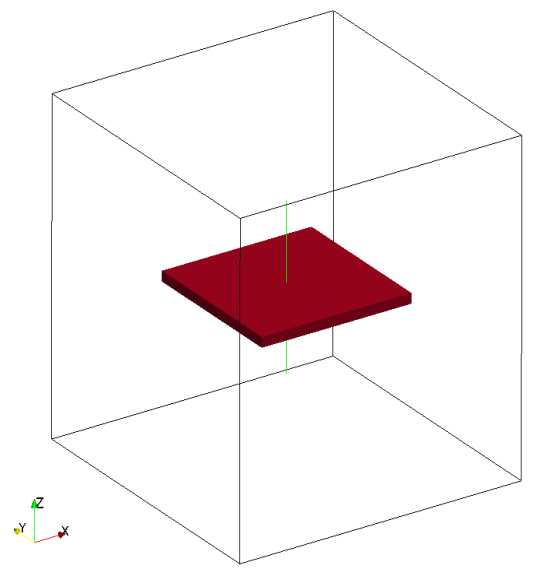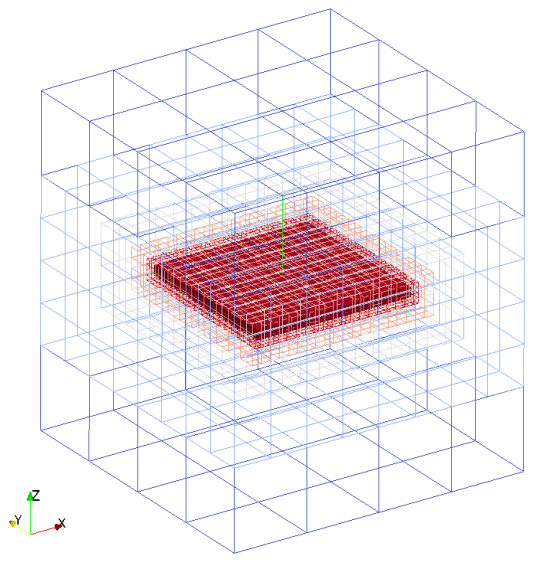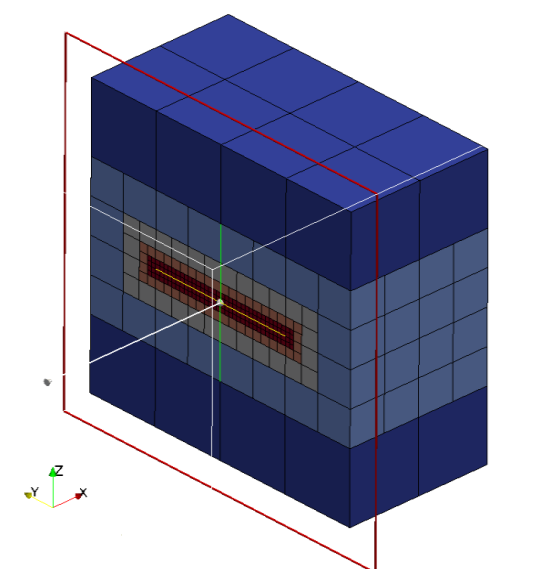# Plane

Planes are defined by an origin and two vectors. Internally in the code, planes are converted into two triangles.

vec2____________
/\              |
|               |
|               |
|               |
|               |
|-------------->|
origin      vec1


Defintion:

spatial_object={
attribute = {
kind = 'boundary',
label = 'plane1',
},
geometry = {
kind = 'canoND',
object = {
origin={2.0,-1.0,-1.0},
vec={{0.0,2.0,0.0},
{0.0,0.0,2.0}},
}
}
}


Seeder file to generate the mesh with plane is given below:

-- Geometry definition

height =  0.41
length = height
-- Use this file as template. Do not modify this file for running some testcases

-- Location to write the mesh in
-- Note the trailing path seperator, needed, if all mesh files should be
-- directory. This directory has to exist before running Seeder in this
-- case
folder = 'mesh/'

--A minimum level,by which all parts in the computational domain should
--at least be resolved with .Default is 0
minlevel  = 6

--Debug output can be used to output preliminary tree in restart format
--and this restart file can be converted to vtu format by harvester.
debug = {debugMode = true, debugFiles = true, debugMesh = 'debug/'}

-- boundingbox: two entries: origin and length in this
-- order, if no keys are used
bounding_cube = {origin = {-length, -length, -length},
length = length*2.0}

-- Each spatial object is defined by an attribute and some geometric
-- entity attached to this attribute. Attribute might be defined mulitple-- times.
-- Attributes are described by a kind (boundary ,seed or Refinement)
--  a level
spatial_object = {
{ attribute = { kind = 'seed', },

-- Geometric objects might by right now:
-- canoND (point, line, plane or box)
-- STL
geometry = { kind = 'canoND',
object = { origin = { 0.0, 0.0, 0.0 },
}
} -- geometry
}, -- seed
{

-- Four edges bounding the plane (east, west, north and south)
-- this is for the lable' east'
attribute = {
kind = 'boundary',
label='east'
},

-- for define the box object requires the origin and vector
geometry = {
kind = 'canoND',
object = {
origin = {length*0.5, -length*0.5, -length*0.5},
vec = {{0.0, length, 0.},
{0.,0.0, length}}
}
}
},
{

-- this is for label 'west'
attribute = {
kind = 'boundary',
label='west'
},
geometry = {
kind = 'canoND',
object = {
origin = {-length*0.5, -length*0.5, -length*0.5},
vec = {{0.0, length, 0.},
{0.,0.0, length}}
}
}
},
{
-- this is for label 'North'
attribute = {
kind = 'boundary',
label='north'
},
geometry = {
kind = 'canoND',
object = {
origin = {-length*0.5, height*0.5, -length*0.5},
vec = {{length, 0.0, 0.},
{0.,0.0, length}}
}
}
},
{
-- this is for label 'South'
attribute = {
kind = 'boundary',
label='south'
},
geometry = {
kind = 'canoND',
object = {
origin = {-length*0.5,-height*0.5, -length*0.5},
vec = {{length, 0.0, 0.},
{0.,0.0, length}}
}
}
}
}


The mesh generated with plane inside mesh:Cut view of planeExample lua file is available at testsuite/plane/seeder.lua.